# SAT II Math II : Equations Based on Word Problems

## Example Questions

### Example Question #1 : Single Variable Algebra

Which of the following phrases can be written as the algebraic expression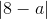?

The absolute value of the difference of a number and eight

Eight decreased by the absolute value of a number

Eight subtracted from the absolute value of a number

The absolute value of the difference of eight and a number

The absolute value of the product of negative eight and a number

The absolute value of the difference of eight and a number

Explanation:is the absolute value of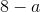, which is the difference of eight and a number. Therefore,is "the absolute value of the difference of eight and a number."

### Example Question #2 : Single Variable Algebra

Which of the following phrases can be written as the algebraic expression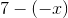?

Seven decreased by the absolute value of a number

The opposite of the difference of seven and a number

The absolute value of the difference of seven and a number

The opposite of a number decreased by seven

Seven decreased by the opposite of a number

Seven decreased by the opposite of a number

Explanation:is seven decreased by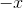, which is the opposite of a number; therefore,is "seven decreased by the opposite of a number."

### Example Question #3 : Single Variable Algebra

Which of the following phrases can be represented by the algebraic expression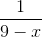?

One divided into the difference of nine and a number

The multiplicative inverse of the difference of nine and a number

Nine decreased by the multiplicative inverse of a number

The multiplicative inverse of the difference of a number and nine

Nine less than by the multiplicative inverse of a number

The multiplicative inverse of the difference of nine and a number

Explanation:is the multiplicative inverse of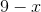, which is the difference of nine and a number. Therefore,is "the multiplicative inverse of the difference of nine and a number".

### Example Question #4 : Single Variable Algebra

Which of the following phrases can be represented by the algebraic expression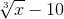Ten decreased by the cube root of a number

Ten less than three times the square root of a number

The cube root of the difference of a number and ten

Ten decreased by three times the square root of a number

Ten less than the cube root of a number

Ten less than the cube root of a number

Explanation:is ten less than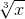, which is the cube root of a number; therefore,is "ten less than the cube root of a number".

### Example Question #1 : Equations Based On Word Problems

Which of the following phrases can be represented by the algebraic expression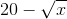Twenty decreased by the square root of a number

The square root of the difference of twenty and a number

The square root of the difference of a number and twenty

Twenty less than the square root of a number

Negative twenty multiplied by the square root of a number

Twenty decreased by the square root of a number

Explanation:is twenty decreased by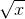, which is the square root of a number, sois "twenty decreased by the square root of a number".

### Example Question #1 : Solve Problems Leading To Two Linear Equations: Ccss.Math.Content.8.Ee.C.8c

Adult tickets to the zoo sell for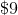; child tickets sell for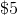. On a given day, the zoo sold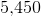tickets and raised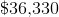in admissions. How many adult tickets were sold?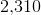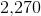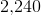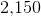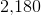Explanation:

Letbe the number of adult tickets sold. Then the number of child tickets sold is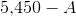.

The amount of money raised from adult tickets is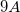; the amount of money raised from child tickets is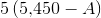. The sum of these money amounts is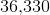, so the amount of money raised can be defined by the following equation: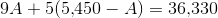To find the number of adult tickets sold, solve for: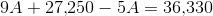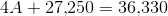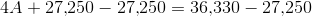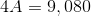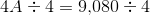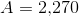adult tickets were sold.

### Example Question #6 : Equations Based On Word Problems

Sarah sells lemonade at the concession stands.  She charges fifty cents per cup of lemonade, and twenty five cents for refills.  What is the equation that represents the total that she will make from the lemonade stand using the variables cupsand refills?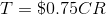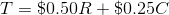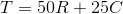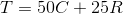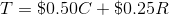Explanation:

Sarah charges fifty cents per cup of lemonade: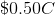Sarah charges twenty five cents for refills: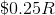Set up the equation by adding the totals.

The answer is: